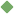calculation 1. [ noun ] (mathematics) the procedure of calculating; determining something by mathematical or logical methods Synonyms: computation computing Related terms: procedure mathematical_process number_crunching transposition recalculation calculate calculate To share this definition press "text" (Facebook, Twitter) or "link" (blog, mail) then paste text link 2. [ noun ] (mathematics,psychology) problem solving that involves numbers or quantities Synonyms: figuring reckoning computation Related terms: problem_solving estimate conversion extrapolation derived_function integral interpolation calculate account 3. [ noun ] (psychology) planning something carefully and intentionally Synonyms: deliberation Examples: "it was the deliberation of his act that was insulting" Related terms: planning forecast Similar spelling: calculating   calculate_on   calculatingly   calculative   calculate   calculator   calculated   calculous   calyculate   calculable   calceolaria   calculus   calycular   callously   caligula   calicular